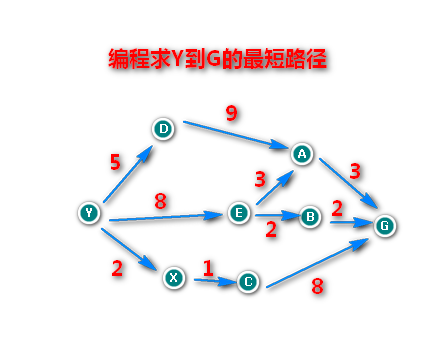# 使用动态规划求Y到G的最短路径解决方案

### 使用动态规划求Y到G的最短路径解决方案```"""

"""
class Node:
def __init__(self,name,prenodes):   # prenodes = {a:10,b:20,c:30},a,b,c也是节点
"""prenodes是它的前趋节点字典"""
self.name = name                # 节点的名字
self.prenodes = prenodes

def f(node):
"""node:一个节点"""
if node.prenodes:          # 如果它有前置节点
dist = []
for n in node.prenodes: # 遍历它的所有前置节点
dist.append( f(n)+node.prenodes[n] ) #
return min(dist)
else:
return 0

y = Node('y',{})     # 第一个节点,没有前置节点
d = Node('d',{y:5})  # 这个节点有一个前置节点
e = Node('e',{y:8})
x = Node('x',{y:2})

a = Node('a',{d:9,e:3})
b = Node('a',{e:2})
c = Node('c',{x:1})

g = Node('g',{a:3,b:2,c:8})
print(f(g))

```